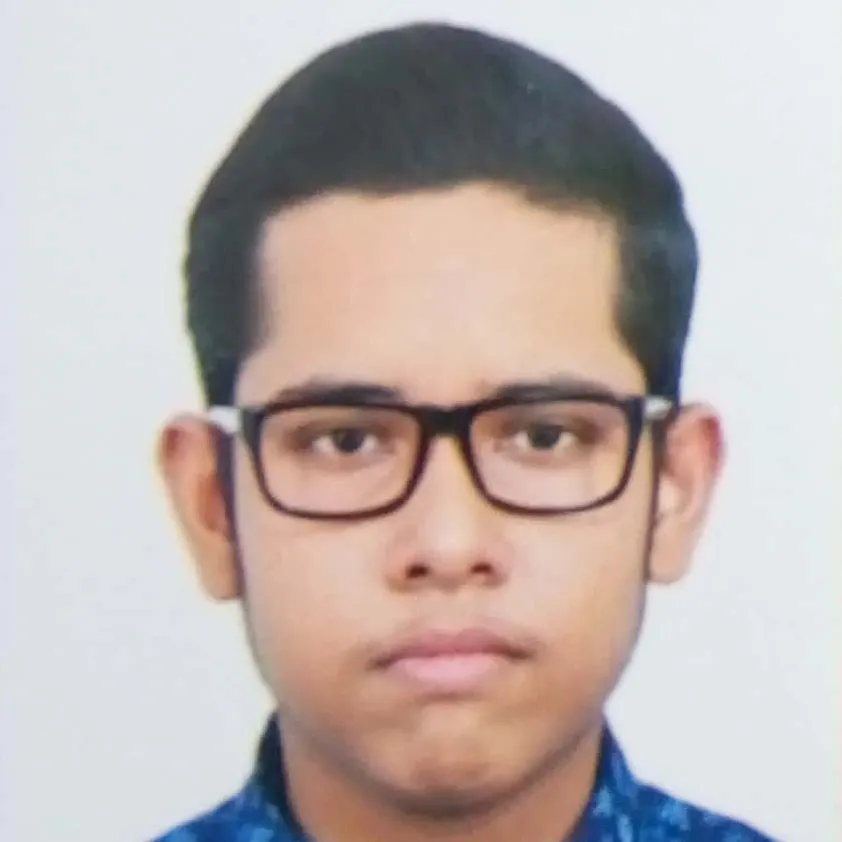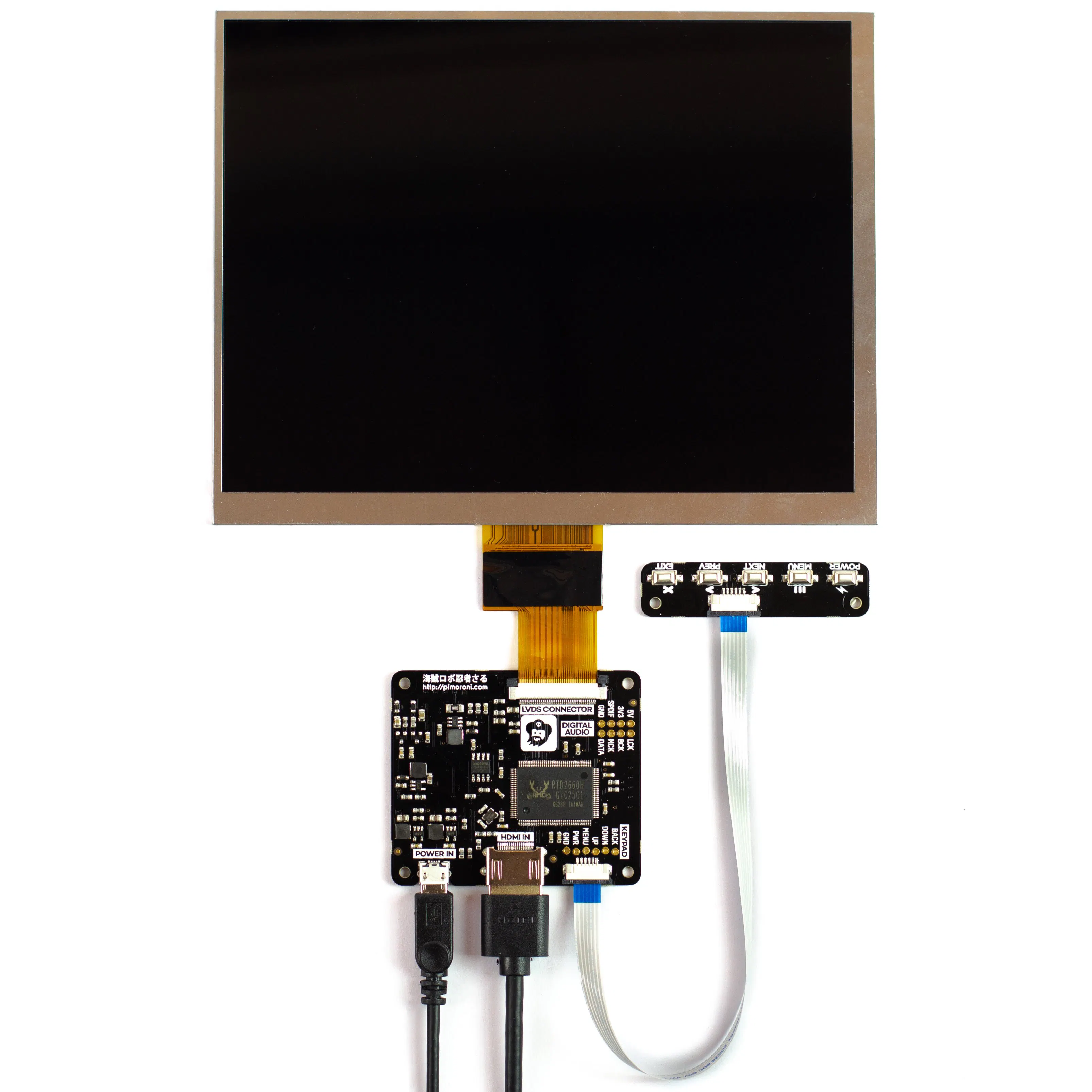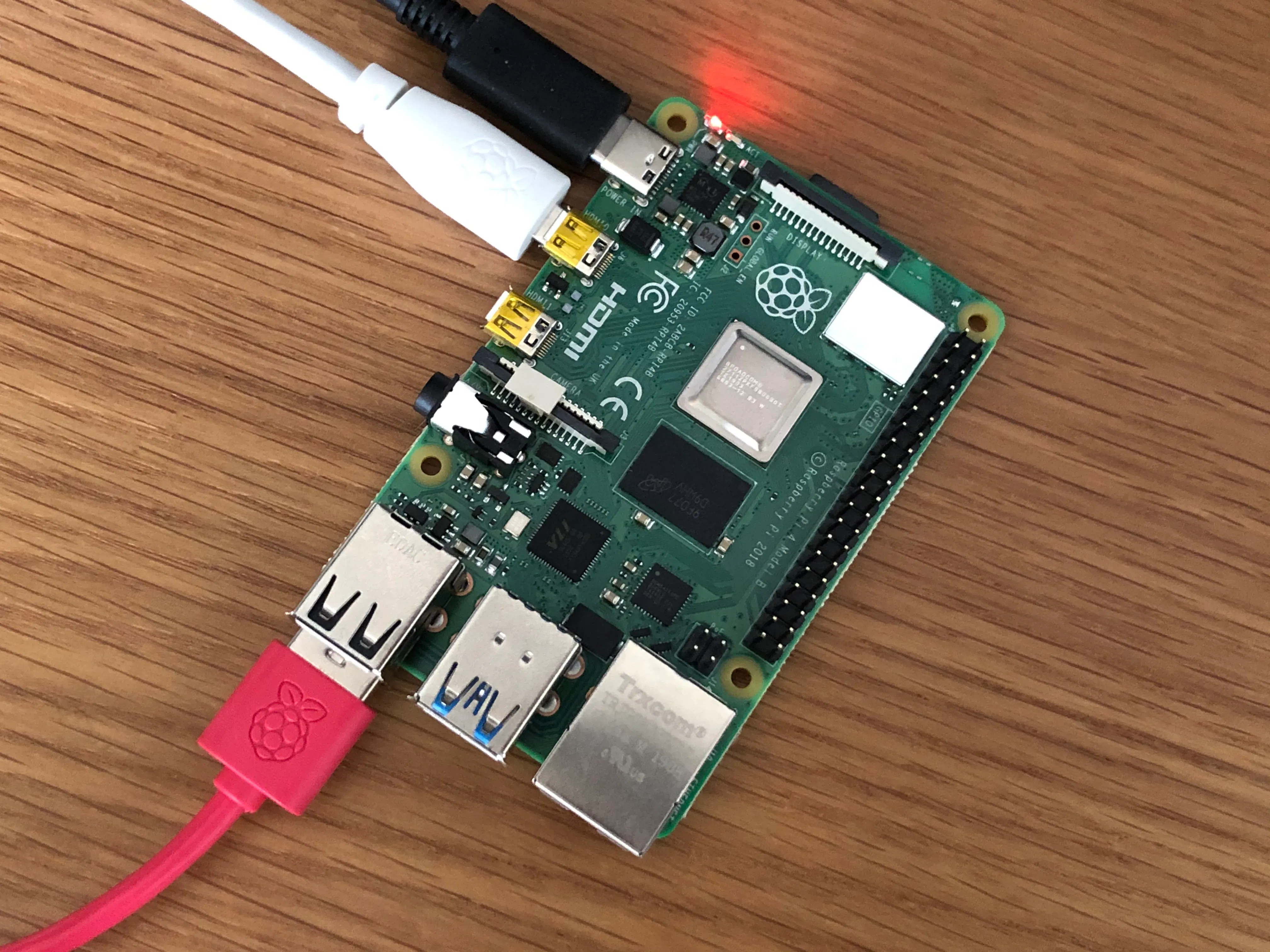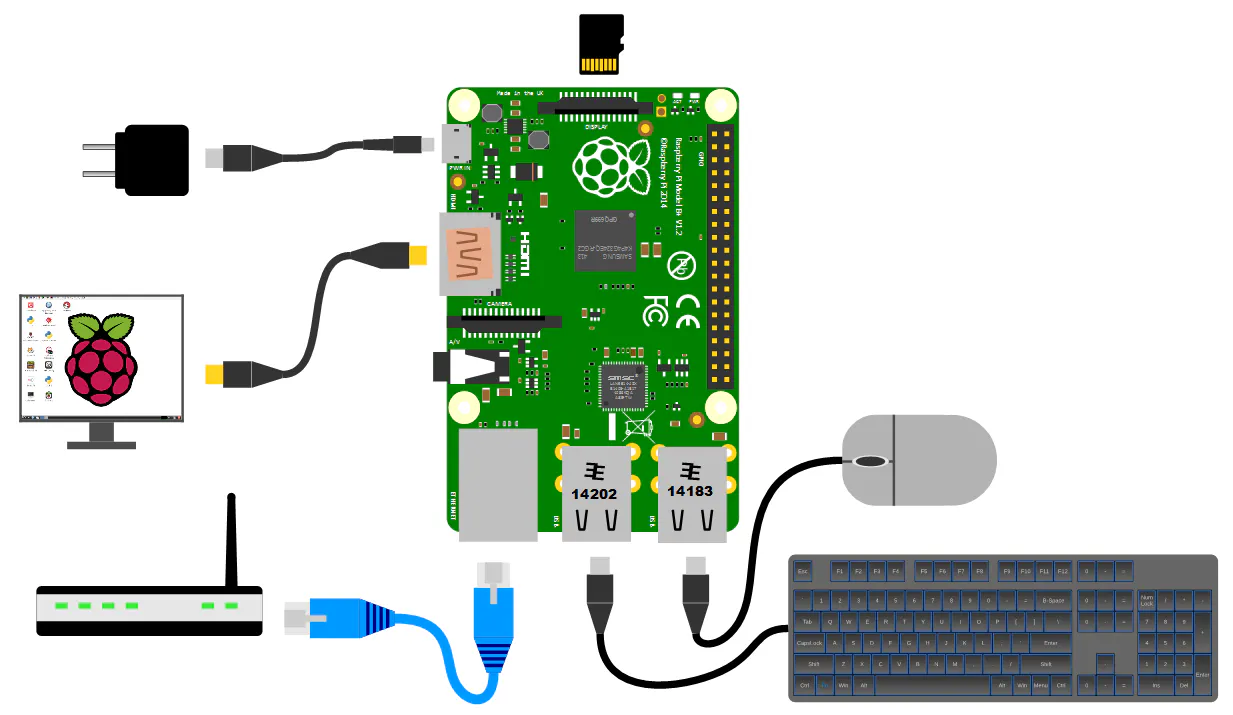# Predictive programming and my conspiracy theory

In this write-up you will explore a world which exists but is kept hidden from us and how the government predicts future using big data.

BeginnerFull instructions provided2 hours10,708## Things used in this project

### Hardware componentsRaspberry Pi 3 Model B
×1Raspberry Pi Keyboard
×1Pimoroni HDMI 8" LCD Screen Kit (1024x768)
×1

## Custom parts and enclosures

### Raspberry pi## Schematics

### Raspberry pi setup for coding## Code

### Weather prediction using Linear regression

Python
Write this code in Google colab for best results , as I wrote it in Google colab
```from google.colab import files
import io
import pandas as pd
import numpy as np
import sklearn as sk
from sklearn.linear_model import LinearRegression
import matplotlib.pyplot as plt
datanew = datanew.drop(['Events', 'Date', 'SeaLevelPressureHighInches',
'SeaLevelPressureLowInches'], axis = 1)
datanew = datanew.replace('T', 0.0)
datanew = datanew.replace('-', 0.0)
datanew.to_csv('mayukhmali_final.csv')
X = data.drop(['PrecipitationSumInches'], axis = 1)
Y = data['PrecipitationSumInches']
Y = Y.values.reshape(-1, 1)
day_index = 798
days = [i for i in range(Y.size)]
clf = LinearRegression()
clf.fit(X, Y)
inp = np.array([, , , , , , , ,
, [29.68], , , , , , , ])
inp = inp.reshape(1, -1)
print('Precipitation for the input is:', clf.predict(inp))
print("Precipitation trend graph: ")
plt.scatter(days, Y, color = 'b')
plt.scatter(days[day_index], Y[day_index], color ='r')
plt.title("Precipitation level")
plt.xlabel("Days")
plt.ylabel("Precipitation in inches")
plt.show()
x_vis = X.filter(['TempAvgF','WindAvgMPH'], axis = 1)
print("Precipitation vs selected attributes graph: ")
for i in range(x_vis.columns.size):
plt.subplot(3, 2, i + 1)
plt.scatter(days, x_vis[x_vis.columns.values[i][:100]],
color = 'b')

plt.scatter(days[day_index],
x_vis[x_vis.columns.values[i]][day_index],
color ='r')
plt.title(x_vis.columns.values[i])
plt.show()
```

## Credits

### MAYUKHMALI DAS

6 projects • 10 followers
Currently pursuing Electronics and Telecommunication engineering from Jadavpur university , India .